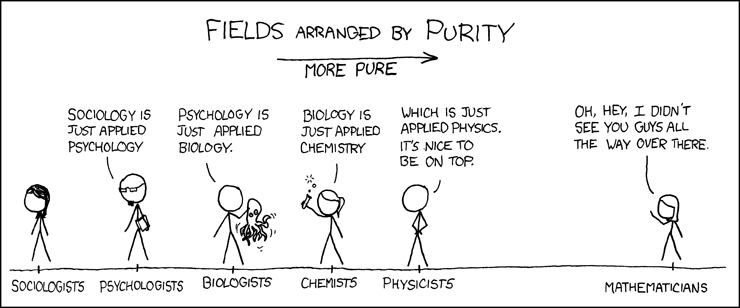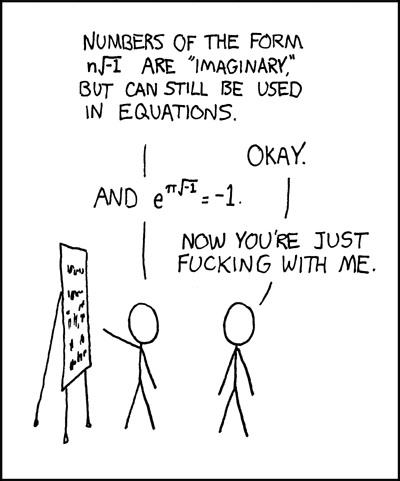You are currently browsing the tag archive for the ‘math’ tag.Euler Formula

The Euler formula, sometimes also called the Euler identity, states$\displaystyle e^{ix}=cosx+isinx,$

where${i}$ is the imaginary unit. Note that Euler’s polyhedral formula is sometimes also called the Euler formula, as is the Euler curvature formula.

The special case of the formula with${x=\pi}$ gives the beautiful identity$\displaystyle e^{i\pi}=-1,$

an equation connecting the fundamental numbers${i,\pi,e,1,}$ and${0}$, the fundamental operations${+}$,${\times}$, and exponentiation, the most important relation${=}$, and nothing else. Gauss is reported to have commented that if this formula was not immediately obvious, the reader would never be a first-class mathematician.